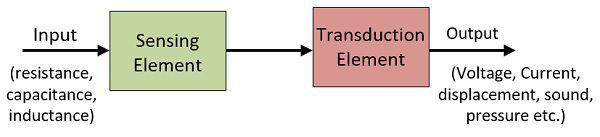# Difference Between Transducer & Inverse Transducer

One of the major difference between the transducer and the inverse transducer is that the transducer changes the non-electrical quantity into an electrical quantity while the inverse transducer changes the electrical quantity into the non-electrical quantity. The other differences between the transducer and inverse transducer are shown below in the comparison chart.

The control action of the physical quantities like flow, rate, position, speed, temperature, pressure etc. depends on the measurement of the physical quantities. In simple words, the control action of the physical quantity is possible only when these quantities are correctly measured.

For measuring the physical quantities, it is essential to convert it into an electrical signal, and this can be done with the help of the transducer. For example, in servomechanism, the position of the shaft is controlled by measuring the exact position of the shaft.

## Comparison Chart

Basis For Comparison Transducer Inverse Transducer
DefinitionThe transducer converts the non-electrical quantity into an electrical quantity.The inverse transducer converts the electrical quantity into the non-electrical quantity.
InputNon-electrical quantityElectrical quantity
Output Electrical quantity Non-electrical quantity
ExamplesPhotoconductive transducer, Thermocouple, Pressure gauge, strain gaugePiezoelectric Transducer, current carrying conductor placed in an magnetic field.

### Definition of Transducer

The transducer is a type of device that converts the physical quantities like pressure, brightness, displacement into an electrical quantity. The process of conversion of quantities is known as the transduction.Examples: The thermocouple converts the temperature into the small voltage. And the LVDT is used for measuring the displacement.

### Definition of Inverse Transducer

The inverse transducer is the transducer which converts the electrical quantity into a non-electrical quantity. In other words, the transducer is a kind of actuator which has an electrical input and the non-electrical output.

Examples: The analogue ammeter and the voltmeter convert the current into a displacement. The oscilloscope is used for converting the electrical signal into a physical displacement.### Key Differences Between Transducer and Inverse Transducer

1. The transducer transforms the non-electrical quantity into the electrical quantity. Whereas, the inverse transducer changes the electrical quantity into the non-electrical quantities.
2. The input of the transducer is the non-electrical quantity. Whereas, the input of the inverse transducer is the electrical quantity.
3. The output of the transducer is the electrical quantity while the output of the inverse transducer is always the non-electrical quantity.
4. The photoconductive cell, thermocouple, pressure gauge are the examples of the non-electrical quantities. The piezoelectrical transducer, current carrying conductor placed in a magnetic field are the examples of the inverse transducer.

### Conclusion

The transducer changes the physical quantity into an electrical quantity. Whereas, the inverse transducer changes the electrical quantity into the physical quantity.